### Home > CALC > Chapter 12 > Lesson 12.1.3 > Problem12-39

12-39.
1. A ball is thrown from a window 25 meters above the ground with an initial velocity of 40 meters per second and an angle of inclination of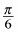. Let the origin be the point on the (level) ground below the window. Homework Help ✎

1. Assume the acceleration due to gravity is −10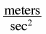. Find x(t) and y(t).

2. Find the angle at which the ball hits the ground.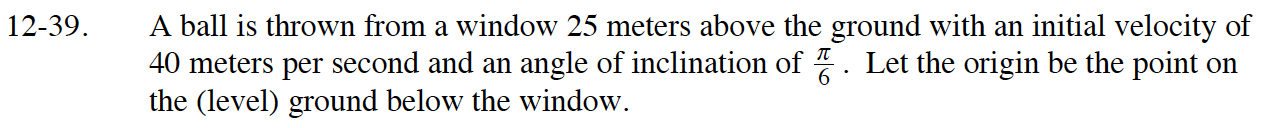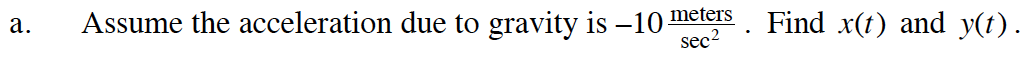$\vec{v}(t)=\langle v_0\cos(\theta),v_0\sin(\theta)+a(t)\rangle=\langle 40\cos\Big(\frac{\pi}{6}\Big),40\sin\Big(\frac{\pi}{6}\Big)-10t\rangle$

$x(t)=40t\cos\Big(\frac{\pi}{6}\Big)+C_1\text{ }y(t)=40t\sin\Big(\frac{\pi}{6}\Big)-5t^2+C_2$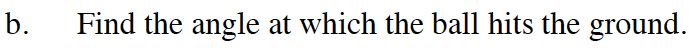First, determine when the ball hits the ground. This is when y(t) = 0.

Then angle is the angle created by the slope.
Think of drawing a slope triangle and calculating the angle.